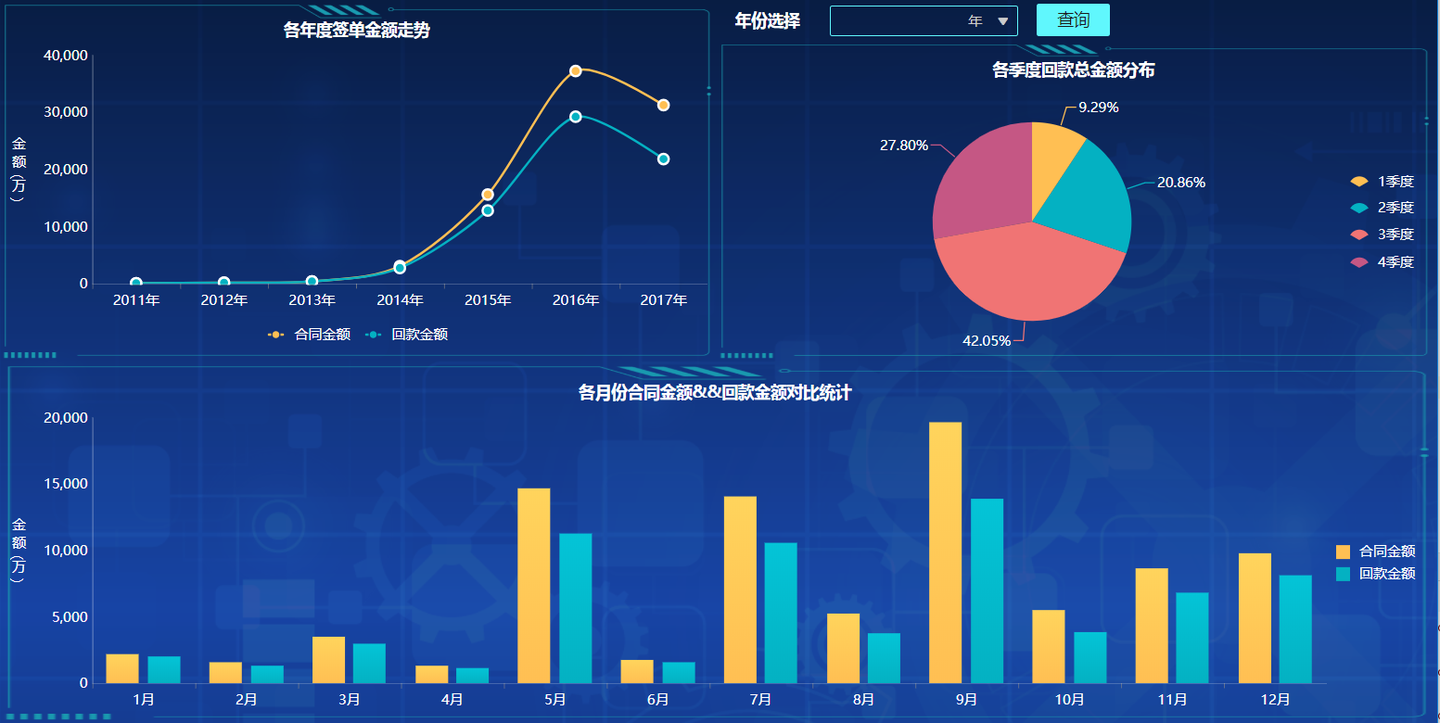1、首先明确领导关注哪些数据和指标

2、用可视化的方式替代传统表格

Python语言简明便捷的特性对我来说还是非常有吸引力的，空想不如行动，为了快速出成果，直接开干。

一、Python数据分析可视化过程

1.DataFrame数据类型转换

# 导入所需要的库
import matplotlib.pyplot as plt
import pandas as pd
import datetime
from pylab import mpl

mpl.rcParams['font.sans-serif'] = ['SimHei'] # 指定默认字体：解决plot不能显示中文问题
mpl.rcParams['axes.unicode_minus'] = False # 解决保存图像是负号'-'显示为方块的问题

pdcsv=pd.merge(pdcsv1,pdcsv2,how='left',on=['合同ID','合同ID']) #将销售合同明细表和销售回款明细表数据进行拼接
df = pd.DataFrame(pdcsv) #转为DataFrame格式方便进行数据处理

2.时间类型转换

i=0 #遍历计数器
for a in df['合同签约时间']:
df.set_value(i, '签约年份',int(datetime.datetime.strptime(a,'%Y-%m-%d %H:%M:%S.0').strftime('%Y'))) #格式化合同签约字段，取出合同签约年份
i=i+1
i=0
for a in df['合同签约时间']:
df.set_value(i, '签约季度',(str((int(datetime.datetime.strptime(a,'%Y-%m-%d %H:%M:%S.0').strftime('%m'))-1)//3+1))+'季度') #格式化合同签约字段，取出合同签约季度
i=i+1
i=0
for a in df['合同签约时间']:
df.set_value(i, '签约月份',int(datetime.datetime.strptime(a,'%Y-%m-%d %H:%M:%S.0').strftime('%m'))) #格式化合同签约字段，取出合同签约月份
i=i+1

3.分组统计&&图表绘制

df.to_csv('D:/DEMO/join.csv',encoding='gb2312') #合并明细数据导出为csv文件数据存储

dfgp1=(df['总金额']/10000.0).groupby(df['签约年份']).sum() #年度总签单金额分组统计，并且单位转为万
dfgp2=(df['付款金额']/10000.0).groupby(df['签约年份']).sum() #年度付款总额分组统计，并且单位转为万
plt.subplot(2,2,1) #限定绘图区域为左上方
plt.ylabel('总金额（万）')#显示y轴名称
plt.title('各年度签单总金额走势')#显示统计标题
dfgp1.plot.line(label='签单金额') #年度总签单金额绘图
dfgp2.plot.line(label='回款金额') #年度付款总额绘折线图
plt.legend()#显示图例

dfgp=df.groupby(df['签约季度'])['付款金额'].sum().reset_index() #各季度付款金额分组统计
plt.subplot(2,2,2)#限定绘图区域为左上方
plt.title('各季度回款金额分布')#显示统计标题
plt.pie(dfgp['付款金额'],labels=dfgp['签约季度'],autopct='%1.1f%%')#各季度付款金额绘制饼图

dfgp=df.groupby(['签约月份'])['总金额','付款金额'].sum().reset_index()#各月度签单&&回款分组统计
dfgp['总金额']=dfgp['总金额']/10000 #并且单位转为万
dfgp['付款金额']=dfgp['付款金额']/10000 #并且单位转为万
plt.subplot(3,1,3)#限定绘图区域为最下方
plt.title('各月度签单合同金额&&回款金额对比')#显示统计标题
plt.bar(dfgp['签约月份'],dfgp['总金额'],width=0.4,label='签单金额')#各月度签单&&回款分组统计绘制对比柱状图
plt.bar(dfgp['签约月份']+0.4,dfgp['付款金额'],width=0.4,label='回款金额')#各月度签单&&回款分组统计绘制对比柱状图，0.4为宽度偏移量
plt.ylabel('总金额（万）')#显示y轴名称
plt.legend()#显示图例

plt.show() # 出图

4.Python可视化成果展示

1.可视化图形界面是静态页面，没有动态的界面数据交互，如悬浮提示、图形联动、数据钻取、超链接跳转等等；

2.界面没有筛选器，无法切片呈现过滤部分数据；

3.如果领导看了已有数据之后，针对一些增长或者异常数据无法做进一步深入分析。

4.开发调试还是有一定周期的，说出来不怕大家笑话哈，我为了调这个Python页面耗费了我大半天时间。。。。

1.自助分析

2.加背景加特效

3.最终敲定